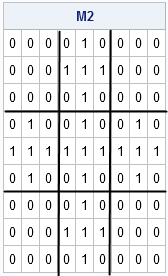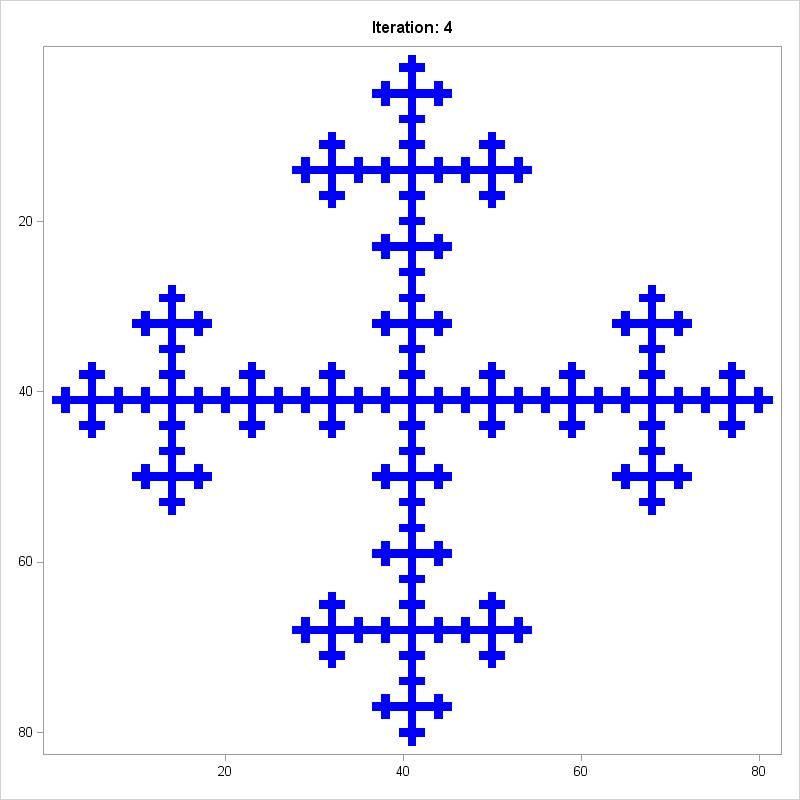I recently posted an article about self-similar structures that arise in Pascal's triangle. Did you know that the Kronecker product (or direct product) can be used to create matrices that have self-similar structure?

The basic idea is to start with a 0/1 matrix and compute a sequence of direct products of the matrix with itself. If M is a matrix that contains only zeros and ones, then M@M is a block matrix where a block of zeros occurs where M has a 0, and a copy of M appears where M has a 1, as shown by the following SAS/IML program:

```proc iml; M = {0 1 0, 1 1 1, 0 1 0}; M2 = M @ M; print M2;```In this example, the original matrix, M, is 3 x 3. The matrix M@M is a 3 x 3 block matrix, where each block is either the zero matrix or a copy of M. If you apply the Kronecker product again and form the matrix M@M@M, you will get a 9 x 9 block matrix, where each block is either the zero matrix or a copy of M.

You can continue this process. The k-fold product of M with itself is a 3k x 3k matrix. Arthur Charpentier provides a nice animation of this iterative process on his Freakonometrics blog, which is where I first read about using the Kronecker product of a 0/1 matrix to create a self-similar structure. If you like to read articles about mathematical aspects of probability and statistics, I highly recommend Charpentier's well-written and always interesting blog.

Printing a 0/1 matrix is not an effective visualization. Instead, I will create a two-color heat map by using the HEATMAPDISC subroutine in SAS/IML 13.1. Because I want to create several heat maps, I wrote the following SAS/IML module that composes a matrix with itself k times and draws a two-color heat map of the result. The following heat map shows the structure of the four-fold Kronecker product M@M@M@M:

```start KroneckerPlot(M, Iters); N = 1; do i = 1 to Iters; N = N @ M; end; call heatmapdisc(N) title="Iteration: "+strip(char(Iters)) colorramp={White Blue} displayoutlines=0 ShowLegend=0; finish;   ods graphics / width=800px height=800px; run KroneckerPlot(M, 4);```Let's say it all together: "Ooooooh!" "Ahhhhh!"

The structures that you can create depend on the arrangement of zeros and ones in the original matrix. Notice that these product matrices get big fast: the k-fold Kronecker product of an n x n matrix is an nk x nk matrix. You might run out of memory if you try to visualize a k-fold product when k or n is greater than 5. The next statements start with a 4 x 4 matrix and display a heat map for the five-fold product, which is a 1024 x 1024 matrix:

```M = {1 1 1 1, 1 1 0 0, 1 0 1 0, 1 0 0 1}; run KroneckerPlot(M, 5); /* 5 iterations of 4x4 matrix ==> 1024 x 1024 matrix */```I call this image "Landing at LaGuardia."

Obviously you can create other structures. You can even generate random structures by using the Bernoulli distribution to create a random 0/1 matrix. I leave you with a few additional statements that create self-similar images. Feel free to create your own matrices. Leave a comment and tell me your favorite matrix for creating a self-similar structure.

```ods graphics / width=500px height=500px; M = {1 1 1 1, 1 0 0 1, 1 0 0 1, 1 1 1 1}; run KroneckerPlot(M, 4);   /* Make random fractal plots! */ call randseed(54321); do i = 1 to 5; M = randfun({4 4}, "Bernoulli", 0.75); run KroneckerPlot(M, 3); end;```
Share